The SGPLOT Procedure

# Overview

The SGPLOT procedure creates one or more plots and overlays them on a single set of axes. You can use the SGPLOT procedure to create statistical graphics such as histograms and regression plots, in addition to simple graphics such as scatter plots and line plots. Statements and options enable you to control the appearance of your graph and add additional features such as legends and reference lines.

The SGPLOT procedure can create a wide variety of plot types, and can overlay plots together to produce many different types of graphs. Examples of Graphs that Can Be Generated by the SGPLOT Procedure contains some examples of graphs that the SGPLOT procedure can create.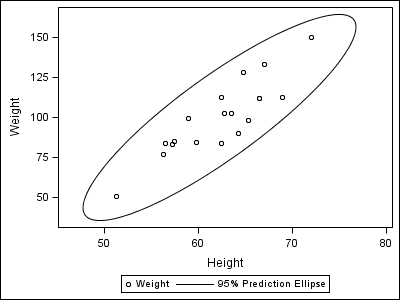The following code creates an ellipse plot: ```proc sgplot data=sashelp.class; scatter x=height y=weight; ellipse x=height y=weight; run;```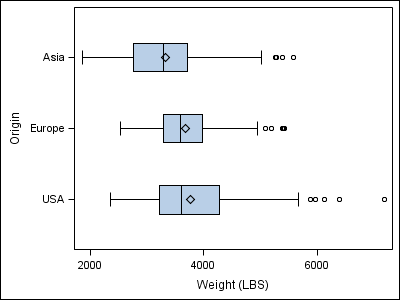The following code creates a horizontal box plot: ```proc sgplot data=sashelp.cars; hbox weight / category=origin; run;```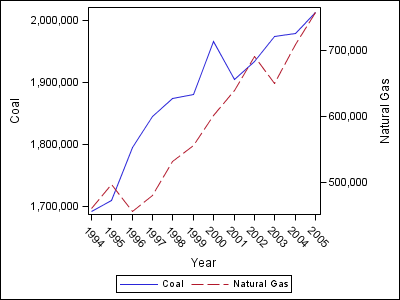The following code creates a graph with two series plots: ```proc sgplot data=sashelp.electric( where=(customer="Residential")); xaxis type=discrete; series x=year y=coal; series x=year y=naturalgas / y2axis; run;```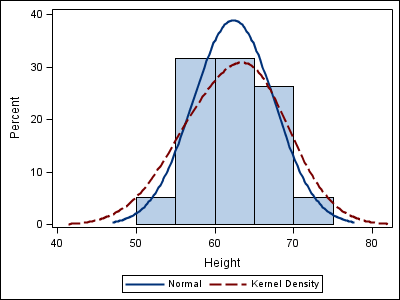The following code creates a graph with a histogram, a normal density curve, and a kernel density curve: ```proc sgplot data=sashelp.class; histogram height; density height; density height / type=kernel; run;```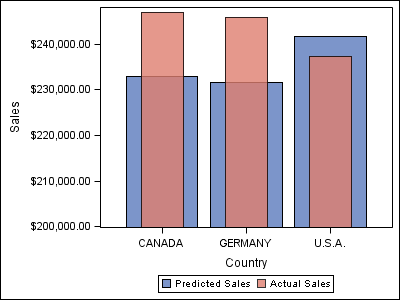The following code creates a graph with two bar charts: ```proc sgplot data=sashelp.prdsale; yaxis label="Sales" min=200000; vbar country / response=predict; vbar country / response=actual barwidth=0.5 transparency=0.2; run;```Previous Page | Next Page | Top of Page